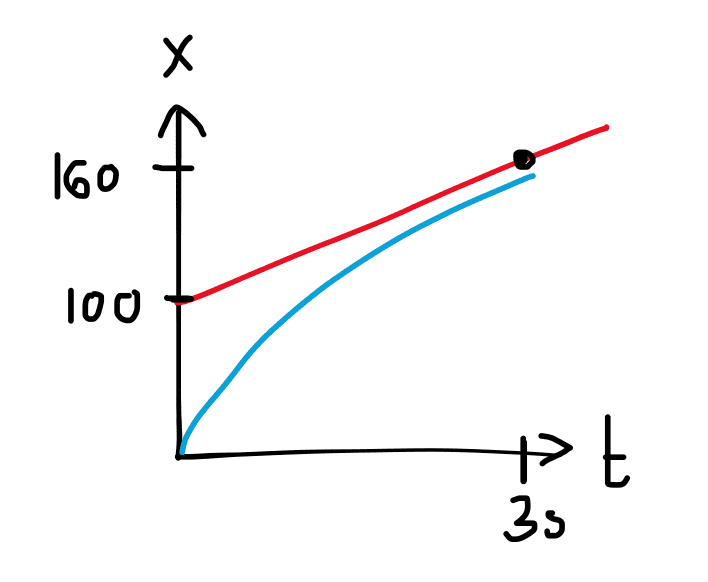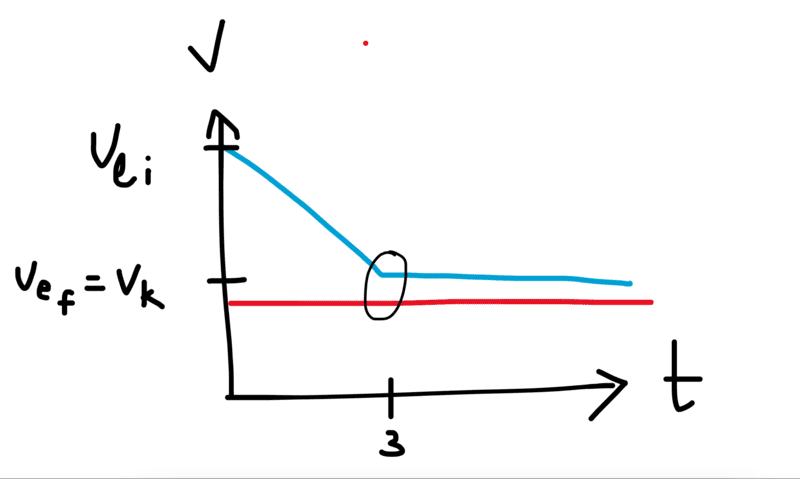# Acceleration required for ship to avoid crashing into another ship

crememars
Homework Statement:
The Starship Enterprise returns from warp drive to ordinary
space with a forward speed of 50 km/s. To the crew’s great sur- prise, a Klingon ship is 100 km directly ahead, traveling in the same direction at a mere 20 km/s. Without evasive action, the Enterprise will overtake and collide with the Klingons in just slightly over 3.0 s. The Enterprise’s computers react instantly to brake the ship. What magnitude acceleration does the Enterprise need to just barely avoid a collision with the Klingon ship? Assume the acceleration is constant.
Relevant Equations:
vf^2 = vi^2 + 2at
xf = xi + vtI drew a x(t) graph to try to map out what was going on. I guessed that in order to just barely avoid the crash, the velocity of Enterprise (v.e) will have to decrease to match the velocity of Klingon (v.k). So v.e final = v.k

Since we're looking for the acceleration, I used this formula:

vf^2 = vi^2 + 2a∆x
v.k^2 = v.e^2 + 2a∆x

for ∆x, I looked for the position of Klingon at t = 3 s.

xf = xi + vt
xf = 100 + 20(3)
xf = 160

∆x would then => 160 - 0 = 160 so

20^2 = 50^2 + 2a(160)
a = -6.6 km/s

which is obviously wrong :( the answer is supposed to be 4500 m/s^2

I feel like I'm missing a fundamental understanding of this problem. Will the Enterprise's speed not be equal to the speed of Klingon at t=3s? I'm super confused.

Staff Emeritus
Homework Helper
Gold Member
Homework Statement:: The Starship Enterprise returns from warp drive to ordinary
space with a forward speed of 50 km/s. To the crew’s great sur- prise, a Klingon ship is 100 km directly ahead, traveling in the same direction at a mere 20 km/s. Without evasive action, the Enterprise will overtake and collide with the Klingons in just slightly over 3.0 s. The Enterprise’s computers react instantly to brake the ship. What magnitude acceleration does the Enterprise need to just barely avoid a collision with the Klingon ship? Assume the acceleration is constant.
Relevant Equations:: vf^2 = vi^2 + 2at
xf = xi + vt

View attachment 315790
I drew a x(t) graph to try to map out what was going on. I guessed that in order to just barely avoid the crash, the velocity of Enterprise (v.e) will have to decrease to match the velocity of Klingon (v.k). So v.e final = v.k
How about also drawing a velocity vs. time graph.

On such a graph, what represents distance traveled?
Since we're looking for the acceleration, I used this formula:

vf^2 = vi^2 + 2a∆x
v.k^2 = v.e^2 + 2a∆x

for ∆x, I looked for the position of Klingon at t = 3 s.

xf = xi + vt
xf = 100 + 20(3)
xf = 160

∆x would then => 160 - 0 = 160 so

20^2 = 50^2 + 2a(160)
a = -6.6 km/s

which is obviously wrong :( the answer is supposed to be 4500 m/s^2

I feel like I'm missing a fundamental understanding of this problem. Will the Enterprise's speed not be equal to the speed of Klingon at t=3s? I'm super confused.

crememars
How about also drawing a velocity vs. time graph.

On such a graph, what represents distance traveled?∆x.k = v.k * ∆t = 20 * 3 = 60
∆x.e = (v.k + v.ei)(∆t) / 2 = (20+50)(3) / 2 = 105

I'm confused, is my graph wrong? Shouldn't the ∆x of Enterprise be equal to 160 if its initial position is 0 and it's about to catch up with Klingon?

Staff Emeritus
Homework Helper
Gold Member
View attachment 315792

∆x.k = v.k * ∆t = 20 * 3 = 60
∆x.e = (v.k + v.ei)(∆t) / 2 = (20+50)(3) / 2 = 105

I'm confused, is my graph wrong? Shouldn't the ∆x of Enterprise be equal to 160 if its initial position is 0 and it's about to catch up with Klingon?
That's not necessarily true that Δt = 3 sec.

What you do know is that Δx.e − Δx.k = 100 km . Right ?

So use your expressions for each of those but with Δt in place of the 3 in each. Then solve for Δt .

Once you have a value for Δt, then the graph can also give you the acceleration needed.

•crememars
Homework Helper
Gold Member
There is something fishy about this problem and its purported answer. One can accept the given solution of 4.5 km/s2 and calculate where each ship would be at the exact collision time. If the collision is barely avoided given that acceleration, the ships should be approximately at the same position. They are not; the Klingon ship will still be ahead by about 25 km which is ¼ of the initial separation of the ships.

•crememars
Staff Emeritus
Homework Helper
Gold Member
There is something fishy about this problem and its purported answer. One can accept the given solution of 4.5 km/s2 and calculate where each ship would be at the exact collision time. If the collision is barely avoided given that acceleration, the ships should be approximately at the same position. They are not; the Klingon ship will still be ahead by about 25 km which is ¼ of the initial separation of the ships.
At an acceleration of −4.5 km/s2, the enterprise slows to 20 km/s in 20/3 s. All is well.

•crememars
Gold Member
At an acceleration of −4.5 km/s2, the enterprise slows to 20 km/s in 20/3 s. All is well.
That's a deceleration of over 450 g. I hope the inertial dampers are working.

•crememars
Homework Helper
Gold Member
2022 Award
a = -6.6 km/s

which is obviously wrong :( the answer is supposed to be 4500 m/s^2
You could try an easier problem first. What if the Klingon ship was at rest 100km away and the Enterprise was traveling at 30 km/s?

•crememars
DrJohn
Or you could change the frame of reference you work with. Just use the speed of the Enterprise relative to the Klingon and then assume the Kligon ship is stationary. And calculate the acceleration to get to zero speed over the stated distance. I think that gives the same result as any over complicated method which has the Klingons motoring along without rear view mirrors or sensors.

EDIT: Just re-read PeroK's suggestion above this. We are suggesting the same thing, using different words.

•crememars
Homework Helper
Hi,

@crememars, are you still with us ?

for ∆x, I looked for the position of Klingon at t = 3 s
##3{1\over 3} ## s if you take the ##100## km/s seriously.

That assumption is where things start to go off the rails: the Klingon will have moved on from there by the time the Enterprise is at that point.

Do you understand that this is a two equation in two unknowns problem, with the two unknowns being time and acceleration ?

The limiting case is realized by an acceleration that achieves identical speeds when the distances between the ships is down to zero. Any more is overdoing it and any less means a collision.

So write down expressions for
##\Delta x = x_{\text{Enterprise}} - x_{\text{Klingon}}## in terms of knowns and unknown ##a## and ##t##.
##\Delta v = v_{\text{Enterprise}} - v_{\text{Klingon}}## in terms of knowns and unknown ##a## and ##t##.

then solve ##\Delta x = 0## and ##\Delta v = 0##

And don't worry about the g forces. After all, they also manage to survive "returns from warp drive to ordinary space"##\ ##

•crememars
crememars
Hi,

@crememars, are you still with us ?

##3{1\over 3} ## s if you take the ##100## km/s seriously.

That assumption is where things start to go off the rails: the Klingon will have moved on from there by the time the Enterprise is at that point.

Do you understand that this is a two equation in two unknowns problem, with the two unknowns being time and acceleration ?

The limiting case is realized by an acceleration that achieves identical speeds when the distances between the ships is down to zero. Any more is overdoing it and any less means a collision.

So write down expressions for
##\Delta x = x_{\text{Enterprise}} - x_{\text{Klingon}}## in terms of knowns and unknown ##a## and ##t##.
##\Delta v = v_{\text{Enterprise}} - v_{\text{Klingon}}## in terms of knowns and unknown ##a## and ##t##.

then solve ##\Delta x = 0## and ##\Delta v = 0##

And don't worry about the g forces. After all, they also manage to survive "returns from warp drive to ordinary space"##\ ##
Hi! I'm so sorry for not responding, I was exhausted and I fell asleep. I'll try out some new equations with t and a as unknowns. Thank you!

Homework Helper
There is an approach that can do it without any algebra.

First, change to a reference frame in which the Klingon ship is at rest. Now you have a ship decellerating to a stop over a known distance with a constant but unknown acceleration. But you know its initial velocity and you know the distance. You want to find the decelleration. It would help if you knew the elapsed time (which would have been three seconds, were it not for the decelleration). So let us work on finding the elapsed time.

We could use a short-cut to get the elapsed time directly from the three second no-acceleration figure. But let us do it a slightly longer way...

Observe that average velocity is the mean of initial and final velocity (as long as the acceleration is uniform). You know both the initial and final velocities. You know that the acceleration is uniform. So you can easily calculate the average velocity.

Now you have motion over a known distance at a known average velocity. You can calculate the elapsed time.

Now you have a uniform acceleration from a known initial velocity to a known final velocity over a known elapsed time. So you can calculate the acceleration.

•crememars
Homework Helper
Gold Member
At an acceleration of −4.5 km/s2, the enterprise slows to 20 km/s in 20/3 s. All is well.
Yes, all is well but 20/3 s if more than "just slightly over" 3.0 s. The problem is misleading in that regard. One must accept only one of (a) "the braking time is slightly over 3.0 s"; (b) the collision is "barely" avoided.

Staff Emeritus
Homework Helper
Gold Member
Yes, all is well but 20/3 s if more than "just slightly over" 3.0 s. The problem is misleading in that regard. One must accept only one of (a) "the braking time is slightly over 3.0 s"; (b) the collision is "barely" avoided.
The problem statement does not say "the braking time is slightly over 3.0 s". It says "Without evasive action, the Enterprise will overtake and collide with the Klingons in just slightly over 3.0 s". Of course that information can be obtained from information already given and is not relevant to solving the problem.

The problem can be solved readily by noting that under constant acceleration from 50 km/s to 20 km/s, the average speed is 35 km/s. I mentioned graphing v vs. t only because OP had already done x vs. t. -but then realized that the solution can be obtained from the v vs. t graph.

Well, the thread has been joined by 5 other people, so I'll sign off here.

•jbriggs444
•